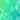# Binary Search

Before we get into the syntax and logic of binary search algorithm, let’s ask ourselves this question. Why do we need binary search at all in the first place?

To understand, let’s work with an example here. Let’s say you were given a name in a letter pad (say ‘Michael’) and you were asked to find that person from a group of 50 people who are standing by name order i.e., Adam stands first, then Alex, then Bob and goes on. You get the idea.

Now, what you do is, pick the 25th person and ask his/her name. Let’s say the person’s name is ‘Monica’. Now you know that ‘Michael’ is somewhere before ‘Monica’ as everyone is standing in ascending order. The reason I picked the 25th person, is coz’ picking that person would give me the max reduction. In the sense, I can ignore the other 25 people after ‘Monica’, since; I know that ‘Michael’ cannot come after ‘Monica’. The idea is filtering 50% of the wrong choices in every question you ask, will give you the fastest route to the destination.

To make it clearer, let’s say I pick a random number (say 35) instead of picking the 25th person. I ask the 35th person, what his/her name is? Now there are two possible outcomes. Either his/her name can be ‘Stuart’ or ‘Henry’. Now, you have a 50 percent chance that you might get either one of these names. If the person says ‘Henry’ then you are lucky, coz’ you only need to filter remaining 15 people to find out your match, but guess what, what if the person’s name is ‘Stuart’, you just got burned here! In other words, instead of filtering from your best bet number, which is 25, now you need to filter from 35.

Hope you get the reason why you need to filter by half every time to find the quickest way to find your match. If all the names in the world are written down together in order and you want to search for the position of a specific name, binary search will accomplish this in a maximum of 35 iterations, which means with asking 35 questions, you will be able to find the person that you are looking for. Isn’t that cool!

Hence, always try to order your array or collection in some way and make sure to index it i.e., if you are adding a row in your excel spreadsheet which you know that the records are gonna increase in the course of time, make sure to add a serial number to each row, so that you can order by ascending/descending anytime to sort the list and, once the list is sorted, perform the binary search to find the record you need.

So, to answer the question ‘why’, binary search algorithm is one of the fastest way to find a random number in a set of indexed numbers, instead of searching the numbers sequentially. Now that’s why!

Alright, funz over. Let’s get to work now. Below is the program to implement binary search in VB Script

Dim testarr(100000)
Dim n, i, res

n = 5643

For i = 1 To 100000
testarr(i) = i
Next

res = CStr(binser(testarr, n))
Wscript.echo res

Function binser(arr, tar)
Dim low, high
low = LBound(arr)
high = UBound(arr)
iter = 0
Do While low <= high
i = Round((low + high) / 2)
If tar = arr(i) Then
binser = True
Wscript.echo iter
Exit Do
ElseIf tar < arr(i) Then
high = i - 1
Else
low = i + 1
End If
iter = iter + 1
Loop
If Not binser Then
binser = False
End If

End Function

### Note:

Binary search works only with sorted arrays, it just won’t work in case of unsorted arrays. Imagine the people are standing in unsorted order in the above example, in which case, you just got double burned! And the odds of finding a person (out of 50) is you gotta ask atmost 49 questions to find the person who you are looking for. You gotta be really lucky otherwise!